International
Tables for
Crystallography
Volume C
Mathematical, physical and chemical tables
Edited by E. Prince

International Tables for Crystallography (2006). Vol. C, ch. 6.3, p. 600

## Section 6.3.1.4. Attenuation (mass absorption) coefficients

E. N. Maslena

aCrystallography Centre, The University of Western Australia, Nedlands, Western Australia 6009, Australia

#### 6.3.1.4. Attenuation (mass absorption) coefficients

| top | pdf |

Since the reduction of intensity depends on the quantity of matter traversed by the beam, the absorption coefficient is often expressed on a mass basis by dividing by the density ρm. μ/ρm defines the attenuation coefficient.

The determination of attenuation coefficients to high precision is possible only when contributions from all different scattering processes are analysed in detail. To a level of accuracy appropriate to most experiments, however, the coefficient can be determined from the atomic cross sections for scattering and photoelectric absorption. Ideally, absorption corrections for scattering from single crystals in the absence of extinction should be evaluated using the Rayleigh cross section for a crystal in the non-reflecting position. However, as Rayleigh scattering is a minor contribution to the total absorption except for the lighter elements, no large error is made by applying the absorption correction appropriate to an assembly of isolated atoms to a single crystal.

Likewise, μ/ρm is, to a good approximation, given by the sum of the attenuation coefficients for each constituent element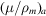, weighted by the mass fraction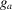for that element, i.e.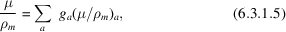where the sum is over the elements. The atomic cross section for attenuation is given by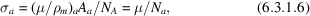where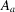is the atomic weight and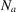is Avogadro's number. The evaluation of the attenuation coefficients is described in Section 4.2.4.